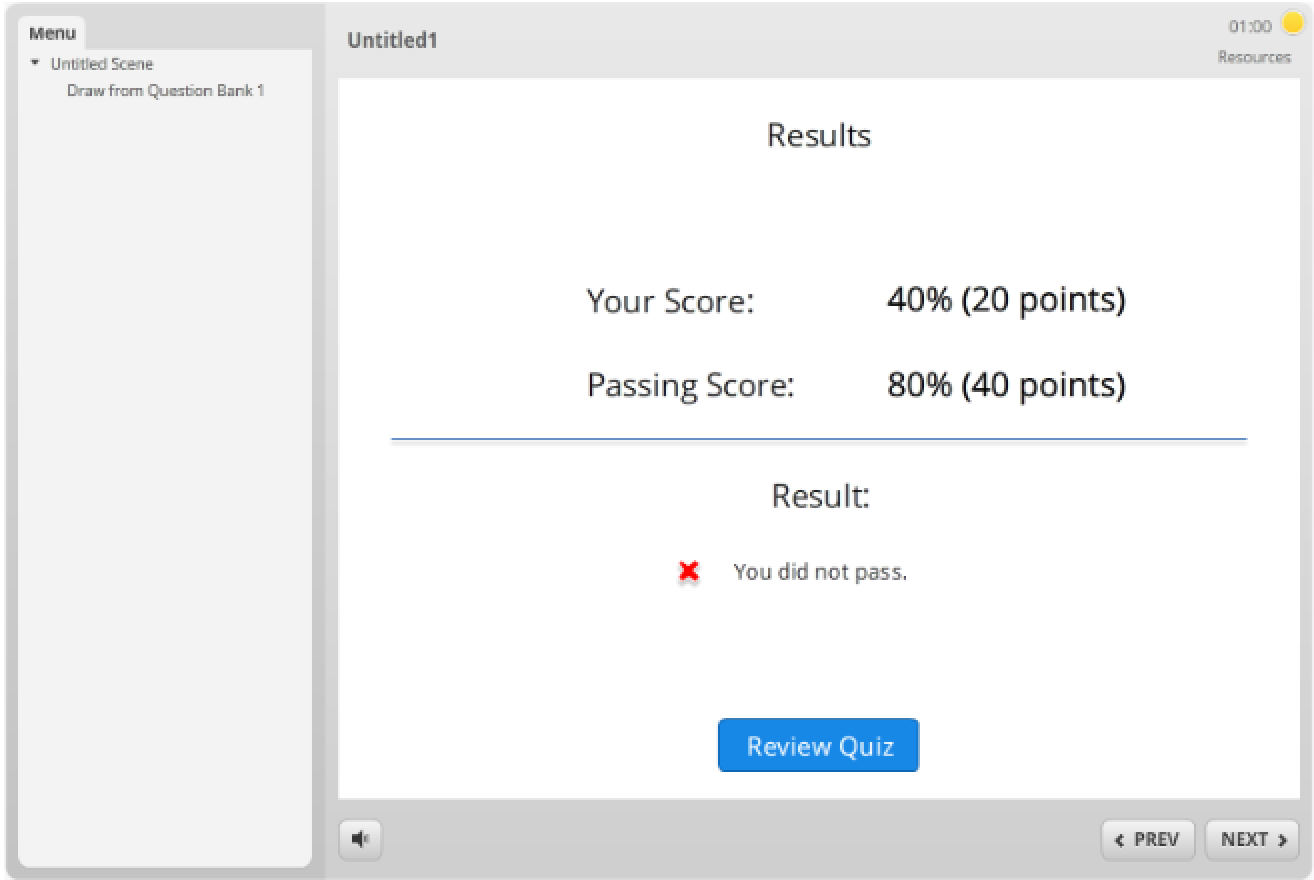# Need help with results slide variables in SL2

Nov 03, 2016

Hi all,

I'm working on a compliance exam, that pulls questions from 12 different question pools. This is a timed exam, so users cannot submit any further answers once the time is up.

On my final result slide, I'd like to show a cumulative score (for all 12 question pools), as well as scores for each of the individual pools.

I've got it *almost* working - the glitch at this point occurs if the exam times out - it seems SL will calculate the cumulative score (%Results1.ScorePoints%) ONLY on questions that have been answered, and not based on the questions answered out of the total number of questions. As such, %Results1.PassPoints% becomes only what the student has attempted, instead of the total number of questions. If a student doesn't get to the questions in the 10, 11, and 12th pool, those seem to be entered as a blank instead of a 0 in the calculations.

I *think* I need to use JavaScript to divide %Results1.ScorePoints% by the total number of questions, instead of just questions attempted, but I don't know enough JS to make that happen.

Alternatively, I'm definitely open to other suggestions other people may have.

thanks,

Melissa

###### 7 RepliesLisa, the issue is that Storyline is NOT counting questions that haven't been answered, not that it's counting them incorrectly.

My exam is out of 55 questions. If a student only answers 23 of the questions, and gets 20 of those 23 correct, Storyline seems to be making the calculation of 20/23 = 87% cumulative score, instead of 20/55 = 36% cumulative score. It should be the latter.

I have the results slide set to calculate results of selected results slides (all of the individual results slides I created for each pool), but if the assessment times out, Storyline will only calculate correct from those questions that were answered. Not calculating correct from ALL of the questions in the exam.I'm not seeing this in my sample test. Scenario Question Bank of 10 Questions set to draw 5 randomly. Quiz Timer set for 1 minute. Each question is 10 points/ea and pass point is 80% or 40/50 points. I answered 2 correctly, then let the timer run out:Do you have a .story file you'd like us to take a look at?The way my course is structured is that I have 12 pools. The exam is set up to pull 55 questions (total) from these 12 pools. To help student metrics, I also have a result slide set up for each pool, so students can see their achievement percentage on each of the topics. The cumulative result slide is set to calculate results from all of the other result slides, with an overall score of 80.

For testing purposes, I've used either 1 minute or 3 minutes to time out. What I'm finding is that if the assessment times out, Storyline does not calculate questions from pools not answered in the cumulative total. (As the example above, if they only complete the first 25 questions, and score 20/25, Storyline is calculating a cumulative result of 80%, instead of 20/55 = 36%.)

It seems that unanswered questions in un-attempted pools are being entered as a blank in the calculations instead of as a zero.